Latest Banking jobs   »

# Reasoning Ability Quiz For Bank Foundation 2023- 13th January

Direction (1-3): Study the following data carefully and answer the questions accordingly.
Mahesh started walking in the east direction from point C. After walking 30 m, he reached point D. From there, he takes a left turn and walks 20 m to reach point E. From E, he turns left and walks 14 m, and reaches point F. Then, he turns left and walks 68 m to reach point G. From point G, he turns right and walks 56 m. Now he reached point H and from there he turns right and walks 80 m to reach the point I. From point I, he turns right and walks 64 m to reach point J.

Q1. I is in which direction with respect to C?
(a) West
(b) South
(c) South East
(d) North West
(e) Can’t be determined

Q2. What is the total distance travelled by Mahesh from point C to point G?
(a) 176 m
(b) 190 m
(c) 132 m
(d) 164 m
(e) None of these

Q3. Point E is in which direction with respect to point I?
(a) East
(b) North West
(c) South East
(d) South West
(e) None of these

Direction (4-5): Study the information given below and answer the questions based on it.
A person walks 20mt from the point M towards South direction and takes a left turn and walks for another 20mt. Then, he takes a left turn and walks for 40mt. After that, he took right turn and walks for 20mt. Then, he turns right and walks for 10mt. He goes for another left turn and walks 50mt and reach to the point N.

Q4. Finally, he is facing in which direction?
(a) North
(b) South
(c) West
(d) East
(e) None of these.

Q5. In which direction is N with respect to his initial position?
(a) South
(b) North-West
(c) North-East
(d) East
(e) None of these.

Direction (6-7): Study the information carefully and answer the questions given below.
A is standing north of B at a distance of 6m. C is standing toward the east of A at a distance of 6m. C is to the north of D and the distance between them is 6m. A is facing north direction, C is facing east, D is facing north and B is facing towards west direction. All of them walk straight for 3m.

Q6. What is the distance between the final position of A and initial position of B?
(a) 10√3m
(b) 9m
(c) 6m
(d) 3√10m
(e) None of the above

Q7. What is the shortest distance between the final position of C and final position of D?
(a) 6√3m
(b) 3√2m
(c) 6m
(d) 6√2m
(e) None of the above

Direction (8-10): Study the information given below and answer the questions based on it.
A person is standing at point M. He starts walking and walks for 5m to the west direction, then he takes a left turn and walks for 8m and reached point N, then he takes a left turn and walks for 4m and reached point O. Point R is 6m to the north of S. Point S is 8m to the west of point P. Point P is 6m to the south of point O.

Q8. What is the shortest distance between point R with respect to point O?
(a) 8m
(b) 12m
(c) 10m
(d) 6m
(e) 14m

Q9. In which of the following direction is point M with respect to point O?
(a) North
(b) North-east
(c) North-west
(d) South-east
(e) South-west

Q10. If point K is 4m to the west of point P, then in which of the following direction is point K with respect to point N?
(a) North
(b) South
(c) North-west
(d) South-east
(e) South-west

Direction (11-12): Study the following data carefully and answer the questions accordingly.
Point P is 10m north of point J. Point K is 15m north of point N. Point J is 15m east of point N. Point M is 6m north of point L, which is 10m east of point K.

Q11. Point L is in which direction of point P?
(a) North
(b) West
(c) South-East
(d) North-West
(e) None of these

Q12. What is the shortest distance between J and K?
(a) 13√5m
(b) 30m
(c) 15√2m
(d) 45m
(e) None of these

Direction (13-15): Study the information given below and answer the questions based on it.
A person is standing at point P. He starts walking towards the west direction and walks for 6m and reached point Q and then he takes a left turn and walks for 4m and reached point R, then he takes a right turn and walks for 3m then he takes a left turn and walks for 4m and reached point T and then he takes another left turn and walks for 3m and reached point M.

Q13. Point O is in south direction of point P in a way that point T, M and O are in a horizontal line, then how far is point O with respect to point M?
(a) 4m
(b) 6m
(c) 5m
(d) 7m
(e) 11m

Q14. In which of the following direction is point M with respect to point P?
(a) South-west
(b) South-east
(c) North
(d) East
(e) North-west

Q15. In which of the following direction is point Q with respect to point T?
(a) North-west
(b) North-east
(c) South-east
(d) North
(e) South-west

Solutions

Solutions (1-3):
Sol.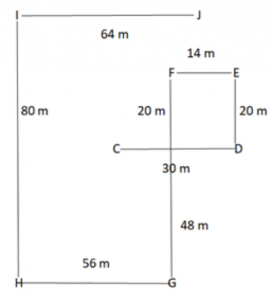S1. Ans. (d)
S2. Ans. (c)
S3. Ans. (c)

Solutions (4-5):
Sol.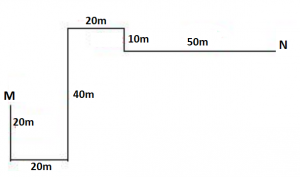S4. Ans. (d)
S5. Ans. (c)

Solutions (6-7):
Sol.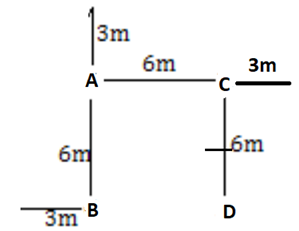S6. Ans. (b)
S7. Ans. (b)

Solutions (8-10):
Sol.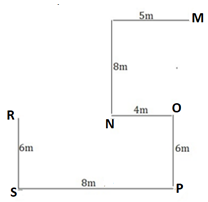S8. Ans. (a)
S9. Ans. (b)
S10. Ans. (b)

Solutions (11-12):
Sol.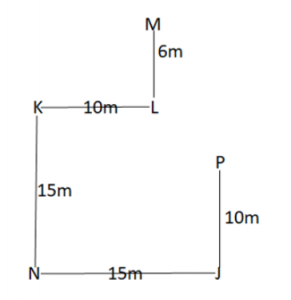S11. Ans. (d)
S12. Ans. (c)

Solutions (13-15):
Sol.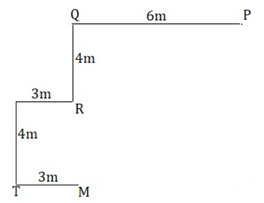S13. Ans. (b)
S14. Ans. (a)
S15. Ans. (b)## FAQs

### What is the selection process of the Bank Clerk?

The selection process of the Bank Clerk is Prelims & Mains.

#### Congratulations!